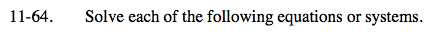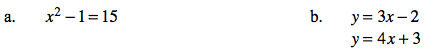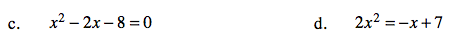Home > AC > Chapter 11 > Lesson 11.1.5 > Problem11-64

11-64.
1. Solve each of the following equations or systems. Homework Help ✎

1. x2 − 1 = 15

2. y = 3x − 2
y = 4x + 3

3. x2 − 2x − 8 = 0

4. 2x2 = −x + 7Add 1 to both sides.

x2 = 16

Solve for x.

x = ± 4

Set the equations equal to each other.

3x − 2 = 4x + 3

Solve for x.Factor the equation using a generic rectangle.

(x − 4)(x + 2) = 0

Solve for x.

Set the equation equal to zero.

Use the quadratic formula.